# GMM-based voice conversion (en)¶

In this notebook, we demonstrate how to build a traditional one-to-one GMM-based voice conversion system. You will learn how to implement voice conversion and how Maximum Likelihood Parameter Generation (MLPG) works though the notebook. Details about GMM-based voice conversion can be found at:

1. Toda, A. W. Black, and K. Tokuda, “Voice conversion based on maximum likelihood estimation of spectral parameter trajectory,” IEEETrans. Audio, Speech, Lang. Process, vol. 15, no. 8, pp. 2222–2235, Nov. 2007.

In this demo, we use differencial spectrum modification technique to get high speech quality described in the following paper:

• Kobayashi, Kazuhiro, et al. “Statistical Singing Voice Conversion with Direct Waveform Modification based on the Spectrum Differential.” Fifteenth Annual Conference of the International Speech Communication Association. 2014.

To run the demonstration notebook, in addition to nnmnkwii and its dependencies, you will need the following packages:

Please make sure that you have all dependneices if you are trying to run the notebook locally.

pip install pysptk pyworld librosa tqdm


Also, please download CMU ARCTIC dataset and configure DATA_ROOT to point where you have data.

:

from os.path import join, expanduser
DATA_ROOT = join(expanduser("~"), "data", "cmu_arctic")
!ls \$DATA_ROOT

cmu_us_awb_arctic  cmu_us_clb_arctic  cmu_us_ksp_arctic  cmu_us_slt_arctic
cmu_us_bdl_arctic  cmu_us_jmk_arctic  cmu_us_rms_arctic


Each sub directiory inside DATA_ROOT must have wav directory.

:

%pylab inline
rcParams["figure.figsize"] = (16,5)

from nnmnkwii.datasets.cmu_arctic import CMUArcticWavFileDataSource
from nnmnkwii.preprocessing.alignment import DTWAligner
from nnmnkwii.preprocessing import trim_zeros_frames, remove_zeros_frames, delta_features
from nnmnkwii.util import apply_each2d_trim
from nnmnkwii.metrics import melcd
from nnmnkwii.baseline.gmm import MLPG

from os.path import basename, splitext
import sys
import time

import numpy as np
from scipy.io import wavfile
from sklearn.mixture import GaussianMixture
from sklearn.model_selection import train_test_split
import pyworld
import pysptk
import librosa
import librosa.display
import IPython
from IPython.display import Audio

Populating the interactive namespace from numpy and matplotlib


## Data¶

In typical one-to-one voice conversion, we need time-aligned source and target speaker’s features. In the demo, we use mel-cepstrum as spectral feature representation and try to convert source speaker’s feature to that of target speaker.

For GMM-based conversion, we need joint feature matrix, which is composed of time-aligned source and target features. Steps to prepare dataset are:

• Compute features for each speaker

• Align features by Dynamic time warping (DTW) for each utterance.

• (GMM voice convertion only) Combine source and target features.

### Data specification¶

:

fs = 16000
fftlen = pyworld.get_cheaptrick_fft_size(fs)
alpha = pysptk.util.mcepalpha(fs)
order = 24
frame_period = 5
hop_length = int(fs * (frame_period * 0.001))
max_files = 100 # number of utterances to be used.
test_size = 0.03
use_delta = True

if use_delta:
windows = [
(0, 0, np.array([1.0])),
(1, 1, np.array([-0.5, 0.0, 0.5])),
(1, 1, np.array([1.0, -2.0, 1.0])),
]
else:
windows = [
(0, 0, np.array([1.0])),
]


### File data sources¶

We need to specify 1) where to find source files and 2) how to process them. In this case,

1. collect_files : Collects .wav files. Also we split the files into train/test set.

2. collect_features : Loads wav file and compute mel-cepstrum.

For CMU ARCTIC case, we can use built-in data source CMUArcticWavFileDataSource to collects wav files. However, you can do it manually pretty easily.

:

class MyFileDataSource(CMUArcticWavFileDataSource):
def __init__(self, *args, **kwargs):
super(MyFileDataSource, self).__init__(*args, **kwargs)
self.test_paths = None

def collect_files(self):
paths = super(
MyFileDataSource, self).collect_files()
paths_train, paths_test = train_test_split(
paths, test_size=test_size, random_state=1234)

# keep paths for later testing
self.test_paths = paths_test

return paths_train

def collect_features(self, path):
x = x.astype(np.float64)
f0, timeaxis = pyworld.dio(x, fs, frame_period=frame_period)
f0 = pyworld.stonemask(x, f0, timeaxis, fs)
spectrogram = pyworld.cheaptrick(x, f0, timeaxis, fs)
spectrogram = trim_zeros_frames(spectrogram)
mc = pysptk.sp2mc(spectrogram, order=order, alpha=alpha)
return mc


We choose clb as source speaker and slt as target speaker, respectively. To align source and target datasets later, define data sources separately.

:

clb_source = MyFileDataSource(data_root=DATA_ROOT,
speakers=["clb"], max_files=max_files)
slt_source = MyFileDataSource(data_root=DATA_ROOT,
speakers=["slt"], max_files=max_files)


### Convert dataset to arrays¶

Since we are using small dataset, it’s enough to load entire dataset into memory at once. To handle variable-length frame lengths, we use PaddedFileSourceDataset here.

:

X = PaddedFileSourceDataset(clb_source, 1200).asarray()
print(X.shape)
print(Y.shape)

(97, 1200, 25)
(97, 1200, 25)


### How data look like?¶

Pick an utterance pair and visualize features.

:

# Plotting util
def plot_parallel(x,y):
figure(figsize=(16,7))
subplot(2,1,1)
librosa.display.specshow(trim_zeros_frames(x).T, sr=fs, hop_length=hop_length, x_axis="time")
colorbar()
subplot(2,1,2)
librosa.display.specshow(trim_zeros_frames(y).T, sr=fs, hop_length=hop_length, x_axis="time")
colorbar()

:

idx = 22 # any
plot_parallel(X[idx],Y[idx])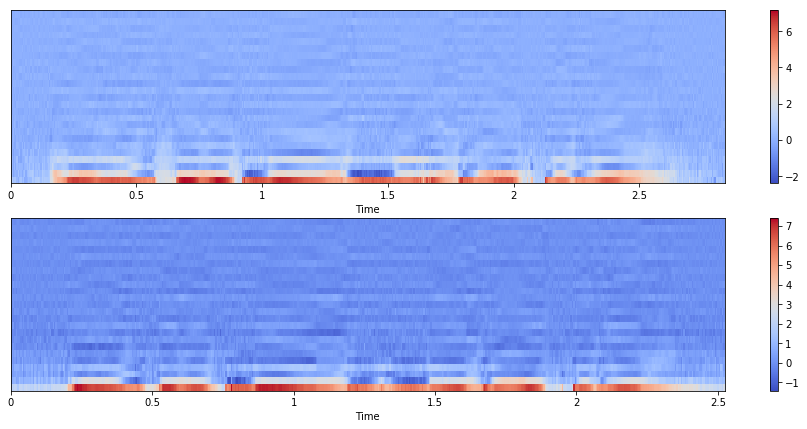### Align source and target features¶

We can use nnmnkwii.preprocessing.DTWAligner

:

# Alignment
X_aligned, Y_aligned = DTWAligner(verbose=0, dist=melcd).transform((X, Y))


### How parallel data look like?¶

:

plot_parallel(X_aligned[idx],Y_aligned[idx])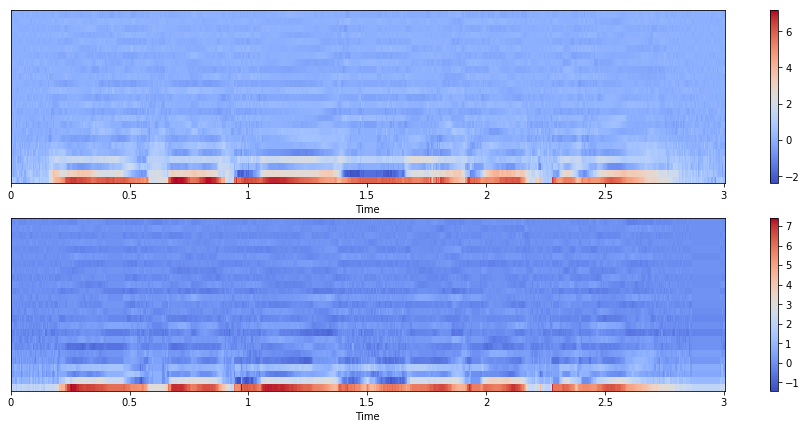0-th mel-cesptrum is a power coefficient by definition. Since we want to focus on spectral characteristics, drop 1-st dimension from aligned features.

:

# Drop 1st (power) dimension
X_aligned, Y_aligned = X_aligned[:, :, 1:], Y_aligned[:, :, 1:]


### Append delta features¶

It’s well known that delta features improve generated speech quality. Later we can use the delta features to sequence-wise parameter generation; a.k.a Maximum Likelihood Parameter Generation (MLPG).

:

static_dim = X_aligned.shape[-1]
if use_delta:
X_aligned = apply_each2d_trim(delta_features, X_aligned, windows)
Y_aligned = apply_each2d_trim(delta_features, Y_aligned, windows)

:

plot_parallel(X_aligned[idx],Y_aligned[idx])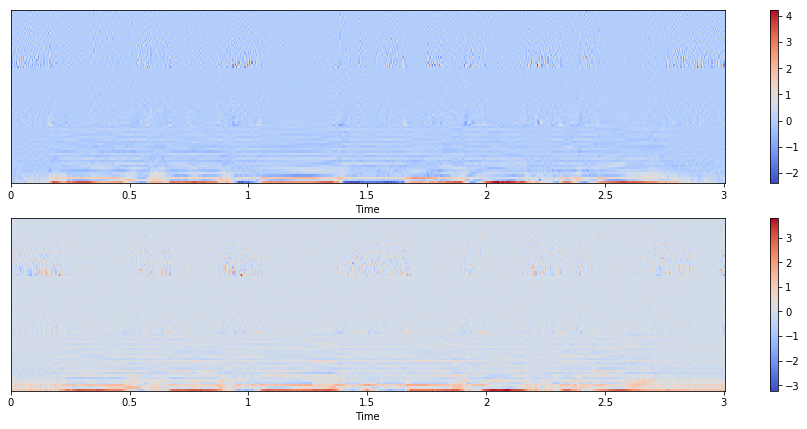### Finally, we get joint feature matrix¶

As we represent features in numpy arrays, it’s very easy to combine them.

:

XY = np.concatenate((X_aligned, Y_aligned), axis=-1).reshape(-1, X_aligned.shape[-1]*2)
print(XY.shape)

(116400, 144)


Note that we padded zeros to data. Removes zeros frames to speed-up training.

:

XY = remove_zeros_frames(XY)
print(XY.shape)

(68194, 144)


## Model¶

We use sklearn.mixture.GaussianMixture. sklearn.mixture.BayesianGaussianMixture can also be used as an drop-in replacement.

:

gmm = GaussianMixture(
n_components=64, covariance_type="full", max_iter=100, verbose=1)

%time gmm.fit(XY)

Initialization 0
Iteration 0
Iteration 10
Iteration 20
Iteration 30
Iteration 40
Iteration 50
Iteration 60
Initialization converged: True
CPU times: user 38min 6s, sys: 3min 23s, total: 41min 29s
Wall time: 10min 32s

:

GaussianMixture(covariance_type='full', init_params='kmeans', max_iter=100,
means_init=None, n_components=64, n_init=1, precisions_init=None,
random_state=None, reg_covar=1e-06, tol=0.001, verbose=1,
verbose_interval=10, warm_start=False, weights_init=None)


### Visualize model¶

Let’s see paramters of GMMs.

#### Means¶

:

for k in range(3):
plot(gmm.means_[k], linewidth=1.5, label="Mean of mixture {}".format(k+1))
legend(prop={"size": 16})

:

<matplotlib.legend.Legend at 0x7f6c670898d0>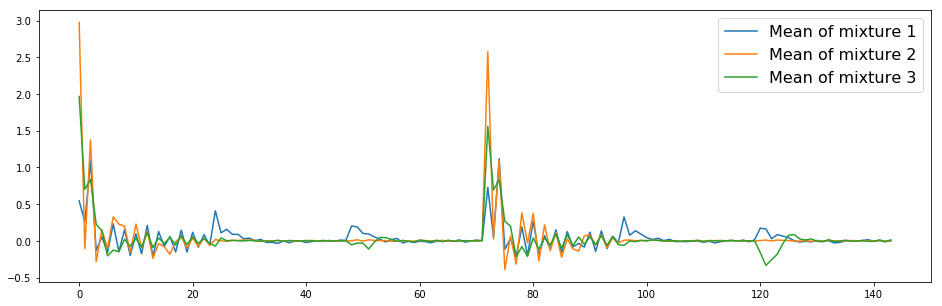#### Covariances¶

:

imshow(gmm.covariances_, origin="bottom left")
colorbar()

:

<matplotlib.colorbar.Colorbar at 0x7f6c67210e80>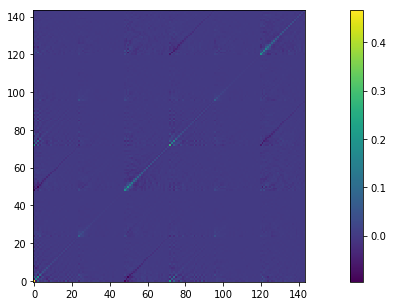Digonal part of covariance matrix, which is expected to be dominant.

:

for k in range(3):
plot(np.diag(gmm.covariances_[k]), linewidth=1.5,
label="Diagonal part of covariance matrix, mixture {}".format(k))
legend(prop={"size": 16})

:

<matplotlib.legend.Legend at 0x7f6c64beff28>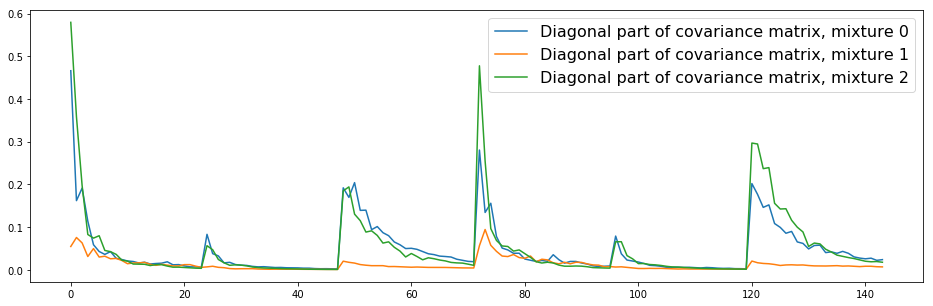## Test¶

Once we trained GMMs, we can perform parameter generation using MLPG.

:

def test_one_utt(src_path, tgt_path, disable_mlpg=False, diffvc=True):
# GMM-based parameter generation is provided by the library in baseline module
if disable_mlpg:
# Force disable MLPG
paramgen = MLPG(gmm, windows=[(0,0, np.array([1.0]))], diff=diffvc)
else:
paramgen = MLPG(gmm, windows=windows, diff=diffvc)

x = x.astype(np.float64)
f0, timeaxis = pyworld.dio(x, fs, frame_period=frame_period)
f0 = pyworld.stonemask(x, f0, timeaxis, fs)
spectrogram = pyworld.cheaptrick(x, f0, timeaxis, fs)
aperiodicity = pyworld.d4c(x, f0, timeaxis, fs)

mc = pysptk.sp2mc(spectrogram, order=order, alpha=alpha)
c0, mc = mc[:, 0], mc[:, 1:]
if use_delta:
mc = delta_features(mc, windows)
mc = paramgen.transform(mc)
if disable_mlpg and mc.shape[-1] != static_dim:
mc = mc[:,:static_dim]
assert mc.shape[-1] == static_dim
mc = np.hstack((c0[:, None], mc))
if diffvc:
mc[:, 0] = 0 # remove power coefficients
b = pysptk.mc2b(mc.astype(np.float64), alpha=alpha)
waveform = engine.synthesis(x, b)
else:
spectrogram = pysptk.mc2sp(
mc.astype(np.float64), alpha=alpha, fftlen=fftlen)
waveform = pyworld.synthesize(
f0, spectrogram, aperiodicity, fs, frame_period)

return waveform


### Listen results¶

Let’s compare the following four different samples. Last two are converted ones. MLPG should avoid temporal discontinuity of parameter sequence and can produce less-noisy speech.

• Source speech

• Target speech

• Converted speech (w/o MLPG)

• Converted speech (w/ MLPG)

:

for i, (src_path, tgt_path) in enumerate(zip(clb_source.test_paths, slt_source.test_paths)):
print("{}-th sample".format(i+1))
wo_MLPG = test_one_utt(src_path, tgt_path, disable_mlpg=True)
w_MLPG = test_one_utt(src_path, tgt_path, disable_mlpg=False)

print("Source:", basename(src_path))
IPython.display.display(Audio(src, rate=fs))
print("Target:", basename(tgt_path))
IPython.display.display(Audio(tgt, rate=fs))
print("w/o MLPG")
IPython.display.display(Audio(wo_MLPG, rate=fs))
print("w/ MLPG")
IPython.display.display(Audio(w_MLPG, rate=fs))

1-th sample
Source: arctic_a0041.wav

Target: arctic_a0041.wav

w/o MLPG

w/ MLPG

2-th sample
Source: arctic_a0036.wav

Target: arctic_a0036.wav

w/o MLPG

w/ MLPG

3-th sample
Source: arctic_a0082.wav

Target: arctic_a0082.wav